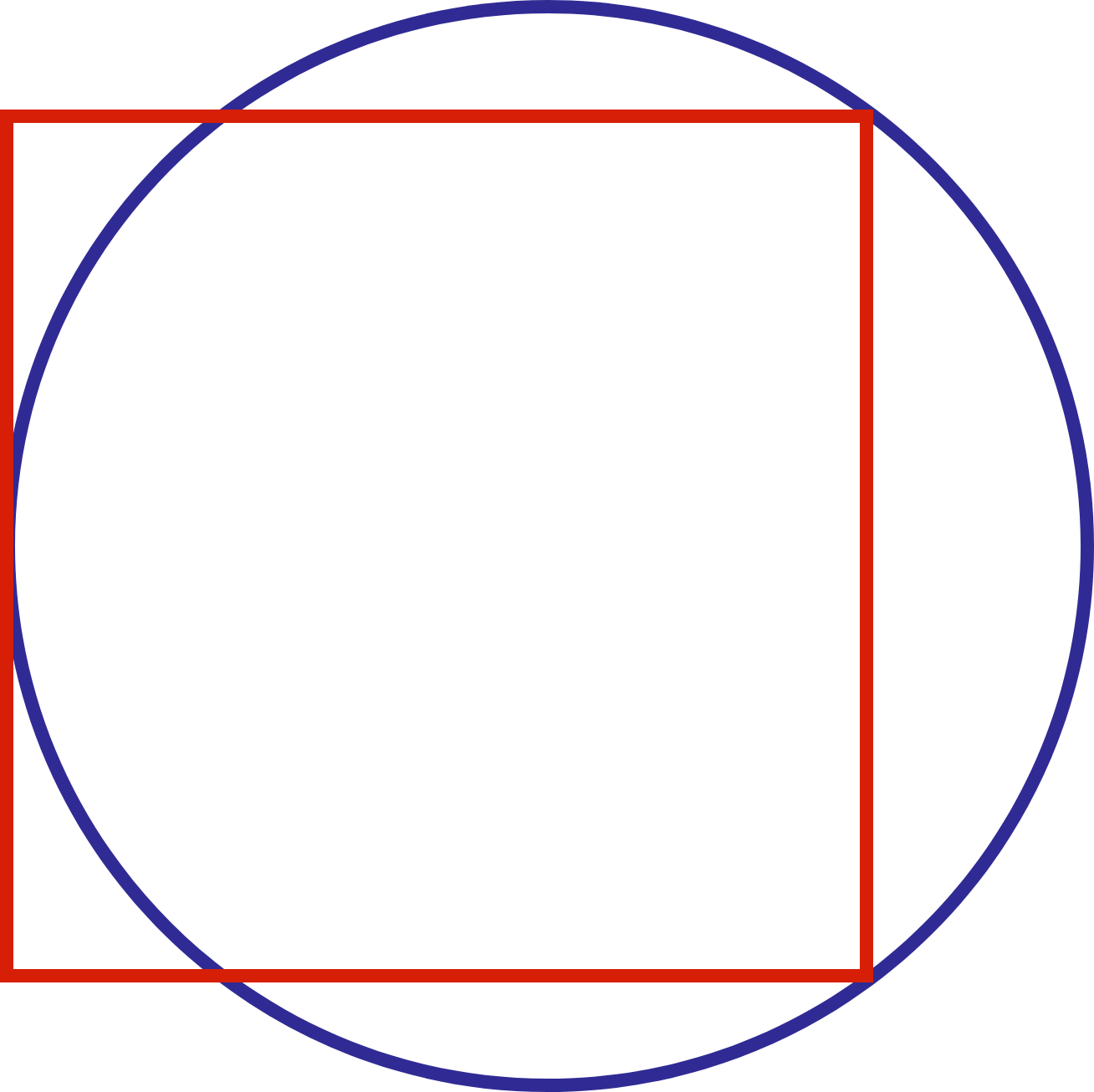# Measuring perimeters

Geometry Level 2In the diagram, the left side of the square is tangent to the circle and two vertices of the square are lying on the circle.

Which has a larger perimeter, the square or the circle?

×